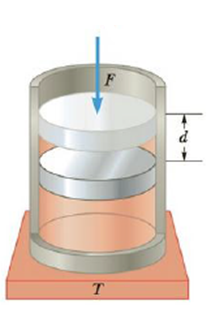Chapter 12, Problem 21P

Chapter
Section
Textbook Problem

An ideal gas is compressed from a volume of Vi = 5.00 L to a volume of Vf = 3.00 L while in thermal contact with a heat reservoir at T = 295 K as in Figure P12.21. During the compression process, the piston moves down a distance of d = 0.130 m under the action of an average external force of F = 25.0 kN. Find (a) the work done on the gas, (b) the change in internal energy of the gas, and (c) the thermal energy exchanged between the gas and the reservoir. (d) If the gas is thermally insulated so no thermal energy could be exchanged, what would happen to the temperature of the gas during the compression?Figure P12.21

(a)

To determine
The work done on the gas.

Explanation

Given Info:

The average external force, F is 25.0kN .

The piston is moved by a distance, d is 0.130m .

Formula to calculate the work done on the gas is,

W=Fd

• F is the average external force
• D is the distance moved by the piston in compression

Substitute 25.0kN for F¯ and 0

(b)

To determine
the change in internal energy of the gas.

(c)

To determine
The thermal energy exchanged between the gas and the reservoir.

(d)

To determine
what will happen to the temperature of the in compression if, the gas is thermally insulated.

Still sussing out bartleby?

Check out a sample textbook solution.

See a sample solution

The Solution to Your Study Problems

Bartleby provides explanations to thousands of textbook problems written by our experts, many with advanced degrees!

Get Started